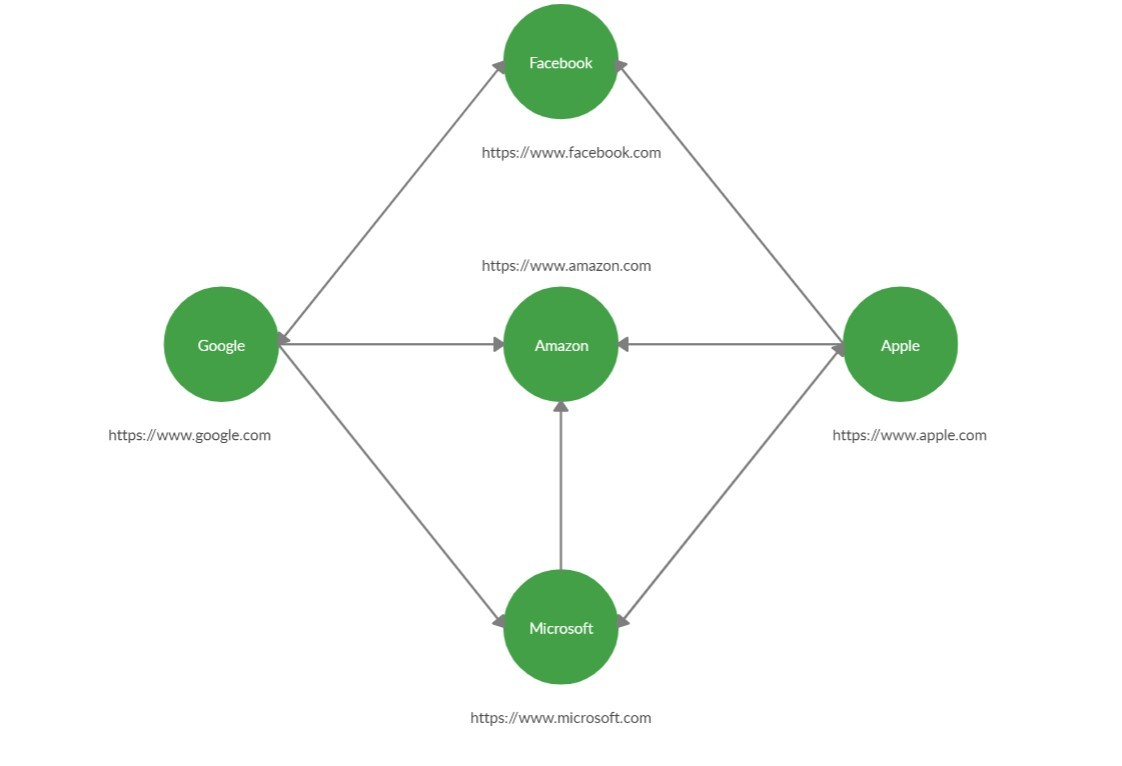# What is a Webcrawler and where is it used?

• Difficulty Level : Hard
• Last Updated : 09 Jul, 2021

Web Crawler is a bot that downloads the content from the internet and indexes it. The main purpose of this bot is to learn about the different web pages on the internet. This kind of bots is mostly operated by search engines. By applying the search algorithms to the data collected by the web crawlers, search engines can provide the relevant links as the response for the request requested by the user. In this article, let’s discuss how the web crawler is implemented.

Webcrawler is a very important application of the Breadth-First Search Algorithm. The idea is that the whole internet can be represented by a directed graph:

• with vertices -> Domains/ URLs/ Websites.
• edges -> Connections.

Example:Approach: The idea behind the working of this algorithm is to parse the raw HTML of the website and look for other URL in the obtained data. If there is a URL, then add it to the queue and visit them in breadth-first search manner.

Note: This code will not work on an online IDE due to proxy issues. Try to run on your local computer.

## Java

 `// Java program to illustrate the WebCrawler`   `import` `java.io.BufferedReader;` `import` `java.io.InputStreamReader;` `import` `java.net.URL;` `import` `java.util.HashSet;` `import` `java.util.LinkedList;` `import` `java.util.List;` `import` `java.util.Queue;` `import` `java.util.regex.Matcher;` `import` `java.util.regex.Pattern;`   `// Class Contains the functions` `// required for WebCrowler` `class` `WebCrowler {`   `    ``// To store the URLs in the` `    ``/ /FIFO order required ``for` `BFS` `    ``private` `Queue queue;`   `    ``// To store visited URls` `    ``private` `HashSet` `        ``discovered_websites;`   `    ``// Constructor for initializing the` `    ``// required variables` `    ``public` `WebCrowler()` `    ``{` `        ``this``.queue` `            ``= ``new` `LinkedList<>();`   `        ``this``.discovered_websites` `            ``= ``new` `HashSet<>();` `    ``}`   `    ``// Function to start the BFS and` `    ``// discover all URLs` `    ``public` `void` `discover(String root)` `    ``{` `        ``// Storing the root URL to` `        ``// initiate BFS.` `        ``this``.queue.add(root);` `        ``this``.discovered_websites.add(root);`   `        ``// It will loop until queue is empty` `        ``while` `(!queue.isEmpty()) {`   `            ``// To store the URL present in` `            ``// the front of the queue` `            ``String v = queue.remove();`   `            ``// To store the raw HTML of` `            ``// the website` `            ``String raw = readUrl(v);`   `            ``// Regular expression for a URL` `            ``String regex` `                ``= ``"https://(\\w+\\.)*(\\w+)"``;`   `            ``// To store the pattern of the` `            ``// URL formed by regex` `            ``Pattern pattern` `                ``= Pattern.compile(regex);`   `            ``// To extract all the URL that` `            ``// matches the pattern in raw` `            ``Matcher matcher` `                ``= pattern.matcher(raw);`   `            ``// It will loop until all the URLs` `            ``// in the current website get stored` `            ``// in the queue` `            ``while` `(matcher.find()) {`   `                ``// To store the next URL in raw` `                ``String actual = matcher.group();`   `                ``// It will check whether this URL is` `                ``// visited or not` `                ``if` `(!discovered_websites` `                         ``.contains(actual)) {`   `                    ``// If not visited it will add` `                    ``// this URL in queue, print it` `                    ``// and mark it as visited` `                    ``discovered_websites` `                        ``.add(actual);` `                    ``System.out.println(` `                        ``"Website found: "` `                        ``+ actual);`   `                    ``queue.add(actual);` `                ``}` `            ``}` `        ``}` `    ``}`   `    ``// Function to return the raw HTML` `    ``// of the current website` `    ``public` `String readUrl(String v)` `    ``{`   `        ``// Initializing empty string` `        ``String raw = ``""``;`   `        ``// Use try-catch block to handle` `        ``// any exceptions given by this code` `        ``try` `{` `            ``// Convert the string in URL` `            ``URL url = ``new` `URL(v);`   `            ``// Read the HTML from website` `            ``BufferedReader be` `                ``= ``new` `BufferedReader(` `                    ``new` `InputStreamReader(` `                        ``url.openStream()));`   `            ``// To store the input` `            ``// from the website` `            ``String input = ``""``;`   `            ``// Read the HTML line by line` `            ``// and append it to raw` `            ``while` `((input` `                    ``= br.readLine())` `                   ``!= ``null``) {` `                ``raw += input;` `            ``}`   `            ``// Close BufferedReader` `            ``br.close();` `        ``}`   `        ``catch` `(Exception ex) {` `            ``ex.printStackTrace();` `        ``}`   `        ``return` `raw;` `    ``}` `}`   `// Driver code` `public` `class` `Main {`   `    ``// Driver Code` `    ``public` `static` `void` `main(String[] args)` `    ``{` `        ``// Creating Object of WebCrawler` `        ``WebCrowler web_crowler` `            ``= ``new` `WebCrowler();`   `        ``// Given URL` `        ``String root` `            ``= ``"https:// www.google.com"``;`   `        ``// Method call` `        ``web_crowler.discover(root);` `    ``}` `}`

Output:

```Website found: https://www.google.com
Website found: https://www.amazon.com
Website found: https://www.microsoft.com
Website found: https://www.apple.com```

Applications: This kind of web crawler is used to acquire the important parameters of the web like:

1. What are the frequently visited websites?
2. What are the websites that are important in the network as a whole?